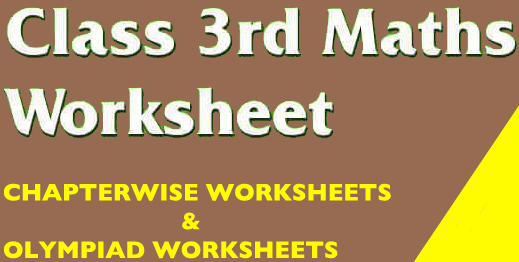CBSE Class 3 Maths Worksheets. Free Printable PDFEvery School those come under the affiliation of Central Board of Schools Education ( CBSE) have one thing in common , and that is they follow the NCERT curriculum and books. The National Council of Education Research and Training ( NCERT ) always focus on designing the curriculum in a planned manner so that students would remain engaged with learning and get acquainted with a variety of important topics.In class 3, the maths curriculum is drafted and designed in an engaging and interesting way. Students are introduced and exposed to various important maths topics in the form of various illustrations and practical examples.
The Syllabus for Class 3 Maths covers topics like addition, subtraction basic geometry, Fun with numbers, Indian Currency, Time and Clock and many more

Class 3 | Printable worksheets | International Mathematics Olympiad (IMO)

CBSE Class 3 Maths Worksheets ( Addition ) – Maths Olympiad Preparation

CBSE Class 3 Maths Worksheets ( Division ) – Maths Olympiad Preparation

CBSE Class 3 Maths Worksheets ( Fraction ) – Maths Olympiad Preparation

CBSE Class 3 Maths Worksheets ( Multiplication ) – Maths Olympiad Preparation

CBSE Class 3 Maths Worksheets ( Place Value ) – Maths Olympiad Preparation

CBSE Class 3 Maths Worksheets ( Subtraction ) – Maths Olympiad Preparation

CBSE Class 3 Maths Worksheets ( Mental Ability ) – Maths Olympiad Preparation

CBSE Class III Mathematics Worksheets – Chapter Wise

CBSE Class 3 Maths Worksheets – Subtraction

CBSE Class 3 Maths Worksheets – Multiplications

CBSE Class 3 Maths Worksheets – Patterns

CBSE Class 3 Maths Worksheets – Data Handling

CBSE Class 3 Maths Worksheets – Division

CBSE Class 3 Maths Worksheets – Geometry

CBSE Class 3 Maths Worksheets – Fun with Numbers

CBSE Class 3 Maths Worksheets – Give & Take

CBSE Class 3 Maths Worksheets – Litres and Millilitres

CBSE Class 3 Maths Worksheets – Measurements

CBSE Class 3 Maths Worksheets – Mental Maths

CBSE Class 3 Maths Worksheets – Patterns

CBSE Class 3 Maths Worksheets – Rupees & Paisa Currency

CBSE Class 3 Maths Worksheets – Shapes and Designs